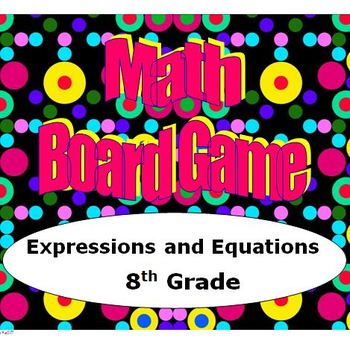# Math Board Game 8th Grade - Expressions and Equations (8.EE)

Rated 4.91 out of 5, based on 15 reviews
15 Ratings;
8th
Subjects
Resource Type
Formats Included
• PDF
Pages
22 pages
Report this resource to TPT

### Description

This board game is fun for the students while they sharpen their skills and deepen their understanding of expressions and equations. They will draw a card and they must correctly answer the question in order to move along the game board. One person will be designated as the Answer Checker. The rules of the game are explained in the Preview.

The questions on the standards in the 8th grade domain Expressions and Equations include:
1. Applying rules of exponents.
2. Solving equations involving square roots and cube roots.
3. Using scientific notation to represent a number in standard form and vice versa.
4. Performing operations using numbers in scientific notation.
5. Finding the slope of a line given a graph, table or equation.
6. Determining the equation of a line given the graph.
7. Solving linear equations in one variable.
8. Determining the solution of a system of equations by using the graph or substitution.

This product includes the following.
1. Game Board
2. 45 Game Cards
3. Game Pieces

The 45 game cards will allow this game to be used for multiple class periods.

I have created a board game for each of the Common Core Domains in 8th grade.

Click below to view the other games.

Math Board Game 8th Grade - The Number System (8.NS)

Math Board Game 8th Grade - Functions (8.F)

Math Board Game 8th Grade - Geometry (8.G)

Math Board Game 8th Grade - Statistics and Probability (8.SP)

The five games are offered together in a bundle.

When purchasing the bundle you will receive all five board games for the price of four games.

Math Board Games Bundle - 8th Grade (8.NS, 8.EE, 8.F, 8.G, 8.SP)

These five games will be a valuable addition to your resources. Each game will provide 2 to 3 days of group activities if you choose.

Thanks for viewing!

Created by Hilda Ratliff
Total Pages
22 pages